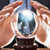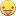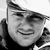# New to Qlik Sense

If you’re new to Qlik Sense, start with this Discussion Board and get up-to-speed quickly.

Announcements
QlikWorld 2023, a live, in-person thrill ride. April 17 - 20, 2023, in Las Vegas! REGISTER TODAY
cancel
Showing results for
Did you mean:Contributor III

## Pick(Match()) as a Variable - Clean up Set Analysis

Hello Fellow Qlikers,

Today I've a got a small challenge that I would like to get some insight into. Essentially, my app works perfectly anyway, but I just would like to clean up my set analysis.

I've got a field select box with certain inline table, and I have a dimension

=\$(=if(GetSelectedCount(Grouping) = 1, Pick(Match((Grouping),
'a',
'b'),
'[a]',
'[b]'),'[a]'))

The actual list of course is more extensive than just a, b. this is where my set analysis gets messy. The reason is that I have quite a large expression that is based on the selected dimension. Let's say for example like this (much shortened version):

Sum({\$<Year={\$(=year(today()))},Cnt={'Y'}>} TOTAL <Month,

//My pick match dimension

\$(=if(GetSelectedCount(Grouping) = 1, Pick(Match((Grouping),
'a',
'b'),
'[a]',
'[b]'),'[a]'))

//Ends here

> Containers)

So my question is, is there a good way to put the whole pick(Match()) section into a variable and use something like \$vGrouping ?

Thanks,

VladLabels (2)

• ### Set Analysis

1 Solution

Accepted SolutionsContributor III
Author

Hi Everyone,

I've actually found a solution I've been looking for myself:

In the front end I have created a Variable:

vGrouping = if(GetSelectedCount(Grouping1)=1,MinString(Grouping1),'dimension')

And now in the expression I just use [\$(vGrouping)], works like a charm. Now at the Back End I can Modify my Grouping1 Table as I want and no need to change anything in the front end or the variable.

Sum({\$<Year={\$(=year(today()))},Cnt={'Y'}>} TOTAL <Month,[\$(vGrouping)]> Containers)

Thanks for everybody's input

Regards,

6 RepliesMaster II
```if(GetSelectedCount(Grouping) = 1, Pick(Match((Grouping),
'b')+1,

Sum({\$<Year={\$(=year(today()))},Cnt={'Y'}>} TOTAL <Month,[a]> Containers) ,

Sum({\$<Year={\$(=year(today()))},Cnt={'Y'}>} TOTAL <Month,[b]> Containers)

))```

Learning never stops.Master II

```if(GetSelectedCount(Grouping) = 1, Pick(Match((Grouping),
'a','b'),

Sum({\$<Year={\$(=year(today()))},Cnt={'Y'}>} TOTAL <Month,[a]> Containers) ,

Sum({\$<Year={\$(=year(today()))},Cnt={'Y'}>} TOTAL <Month,[b]> Containers)

),
Sum({\$<Year={\$(=year(today()))},Cnt={'Y'}>} TOTAL <Month,[a]> Containers)
)```

or

```if(GetSelectedCount(Grouping) = 1, Pick(Match((Grouping),
'b'),

Sum({\$<Year={\$(=year(today()))},Cnt={'Y'}>} TOTAL <Month,[b]> Containers)

),
Sum({\$<Year={\$(=year(today()))},Cnt={'Y'}>} TOTAL <Month,[a]> Containers)
)```
Learning never stops.Contributor III
Author

@pradosh_thakur  Hi Pradosh, this is not what I am looking for. First of all my Pick(Match()) Dimension list is 25 entries long, secondly I have 7 different expressions most of which require the Pick(Match()) to be used 4-7 times in them. unfortunately it is a sub-optimal suggestionMVP
Is it possible to rethink the logic? It looks to me that you are doing pick(match()) to fetch an fieldname listed in an inline. Can't you just choose the minstring of that field? It would make your expressions a lot easier.

Sum({\$<Year={\$(=year(today()))},Cnt={'Y'}>} TOTAL <Month, [\$(=minstring(Grouping))]> Containers)MVP & Luminary

In addition to the suggestion from Vegar a concat() instead of minstring() might be useful to apply multiple values to the TOTAL.

- MarcusContributor III
Author

Hi Everyone,

I've actually found a solution I've been looking for myself:

In the front end I have created a Variable:

vGrouping = if(GetSelectedCount(Grouping1)=1,MinString(Grouping1),'dimension')

And now in the expression I just use [\$(vGrouping)], works like a charm. Now at the Back End I can Modify my Grouping1 Table as I want and no need to change anything in the front end or the variable.

Sum({\$<Year={\$(=year(today()))},Cnt={'Y'}>} TOTAL <Month,[\$(vGrouping)]> Containers)

Thanks for everybody's input

Regards,